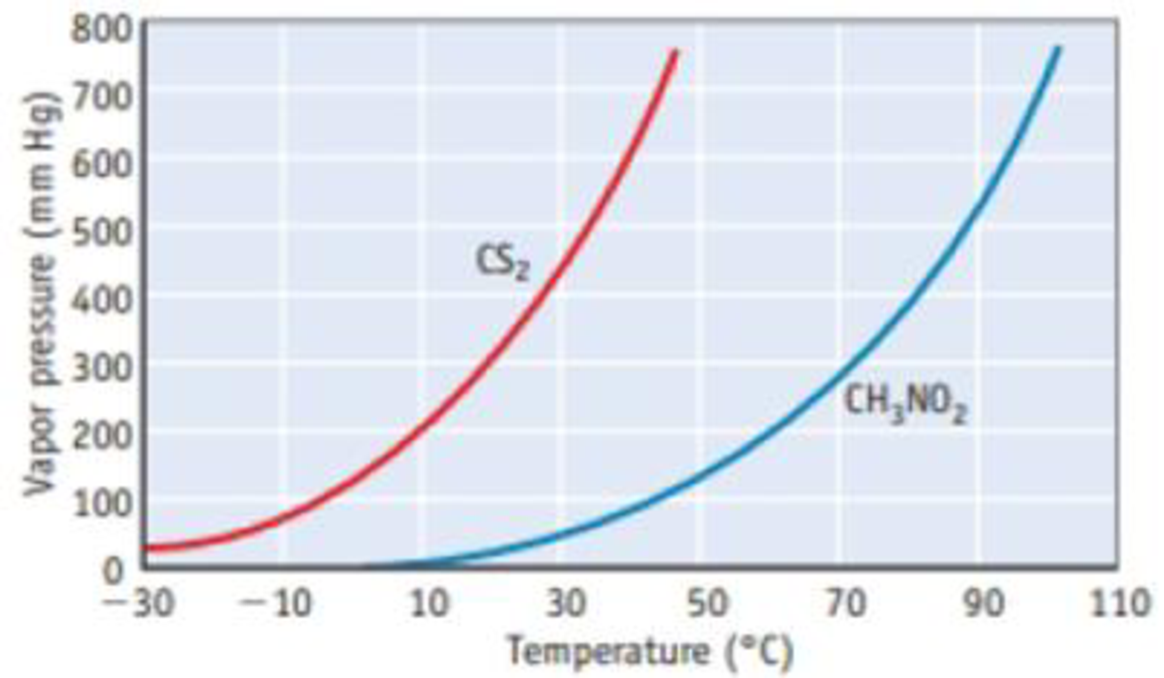Chapter 11, Problem 19PS

Chapter
Section
Textbook Problem

Vapor pressure curves for CS2 (carbon disulfide) and CH3NO2 (nitromethane) are drawn here. (a) What are the approximate vapor pressures of CS2 and CH3NO2 at 40 °C? (b) What type(s) of intermolecular forces exist in the liquid phase of each compound? (c) What is the normal boiling point of CS2? Of CH3NO2? (d) At what temperature does CS2 have a vapor pressure of 600 mm Hg? (e) At what temperature does CH3NO2 have a vapor pressure of 600 mm Hg?ss

(a)

Interpretation Introduction

Interpretation: The approximate vapor pressure curve for CS2 and CH3NO2 at 40oC should be determined

Concept Introduction: Vapor pressure is nothing but the pressure of a vapor in contact with its liquid or solid form.

When a liquid and vapor are in equilibrium the pressure exerted by the vapor is called the equilibrium vapor pressure.

If intermolecular force is small the vapor pressure of the substance is high and the boiling point will be low.

Explanation

The figure is,

From the figure, the vapor pressure of CS2 at 40oC is 620mm

(b)

Interpretation Introduction

Interpretation: From the vapor pressure curve for CS2 and CH3NO2 answer the following questions.

Concept Introduction:

Intermolecular force: The attractive force that withholds two molecules is called as intermolecular force. The influence of intermolecular forces depends on molar mass and the functional group present in the molecule.

Dipole-Dipole Interactions: The attractive force that holds two polar molecules with help of dipole moment present in them is called as Dipole-Dipole Interactions.

The partial positive charge end of one molecule is attracted to the partial negative charge of a neighboring molecule.

Induced Dipole - Induced Dipole Forces: The attractive force that holds two nonpolar molecules with help of temporary dipole moment present in them is called as induced dipole-induced dipole forces.

(c)

Interpretation Introduction

Interpretation: The normal boiling point of CS2 and CH3NO2 should be determined.

Concept Introduction:

Boiling point: It is the temperature at which liquid converts to vapor. At boiling point the vapor pressure of liquid and the pressure of the surroundings are equal.

(d)

Interpretation Introduction

Interpretation: The temperature at which CH3NO2 has a vapor pressure of 600mmHg should be determined.

Concept Introduction:

Vapor pressure is nothing but the pressure of a vapor in contact with its liquid or solid form.

When a liquid and vapor are in equilibrium the pressure exerted by the vapor is called the equilibrium vapor pressure.

(e)

Interpretation Introduction

Interpretation: The temperature at which CS2 has a vapor pressure of 600mmHg should be determined.

Concept Introduction:

Vapor pressure is nothing but the pressure of a vapor in contact with its liquid or solid form.

When a liquid and vapor are in equilibrium the pressure exerted by the vapor is called the equilibrium vapor pressure.

Still sussing out bartleby?

Check out a sample textbook solution.

See a sample solution

The Solution to Your Study Problems

Bartleby provides explanations to thousands of textbook problems written by our experts, many with advanced degrees!

Get Started